# Applications of NLM.pdf

Mar. 22, 2023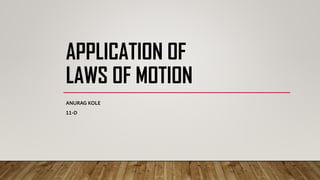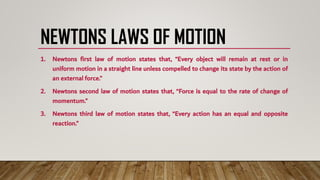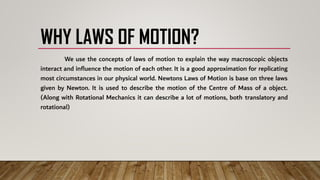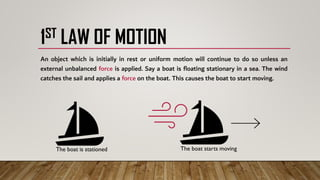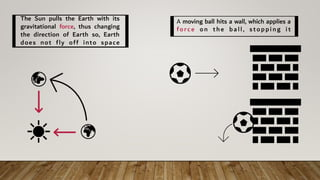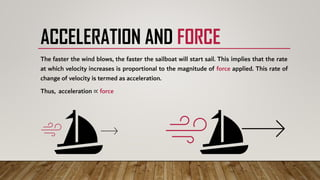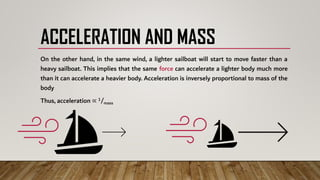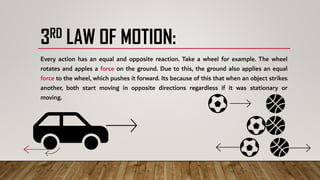1 of 10

### Applications of NLM.pdf

1. APPLICATION OF LAWS OF MOTION ANURAG KOLE 11-D
2. NEWTONS LAWS OF MOTION 1. Newtons first law of motion states that, “Every object will remain at rest or in uniform motion in a straight line unless compelled to change its state by the action of an external force.” 2. Newtons second law of motion states that, “Force is equal to the rate of change of momentum.” 3. Newtons third law of motion states that, “Every action has an equal and opposite reaction.”
3. WHY LAWS OF MOTION? We use the concepts of laws of motion to explain the way macroscopic objects interact and influence the motion of each other. It is a good approximation for replicating most circumstances in our physical world. Newtons Laws of Motion is base on three laws given by Newton. It is used to describe the motion of the Centre of Mass of a object. (Along with Rotational Mechanics it can describe a lot of motions, both translatory and rotational)
4. APPLICATION:
5. 1ST LAW OF MOTION An object which is initially in rest or uniform motion will continue to do so unless an external unbalanced force is applied. Say a boat is floating stationary in a sea. The wind catches the sail and applies a force on the boat. This causes the boat to start moving. The boat is stationed The boat starts moving
6. The Sun pulls the Earth with its gravitational force, thus changing the direction of Earth so, Earth does not fly off into space Α moving ball hits a wall, which applies a force on the ball, stopping i t
7. ACCELERATION AND FORCE The faster the wind blows, the faster the sailboat will start sail. This implies that the rate at which velocity increases is proportional to the magnitude of force applied. This rate of change of velocity is termed as acceleration. Thus, acceleration ∝ force
8. ACCELERATION AND MASS On the other hand, in the same wind, a lighter sailboat will start to move faster than a heavy sailboat. This implies that the same force can accelerate a lighter body much more than it can accelerate a heavier body. Acceleration is inversely proportional to mass of the body Thus, acceleration ∝ 1/mass
9. 3RD LAW OF MOTION: Every action has an equal and opposite reaction. Take a wheel for example. The wheel rotates and apples a force on the ground. Due to this, the ground also applies an equal force to the wheel, which pushes it forward. Its because of this that when an object strikes another, both start moving in opposite directions regardless if it was stationary or moving.
10. THANK YOU THIS IS THE END OF THE PRESENTATION